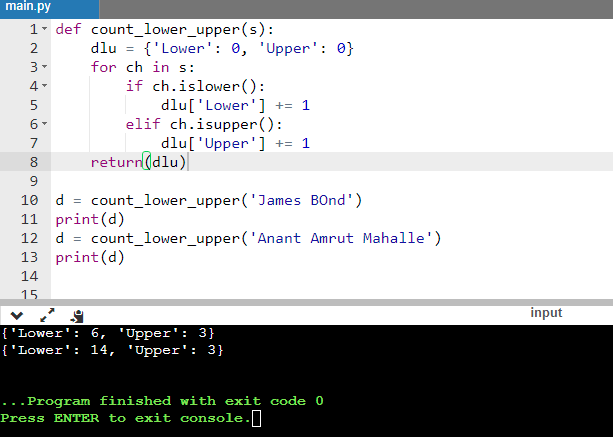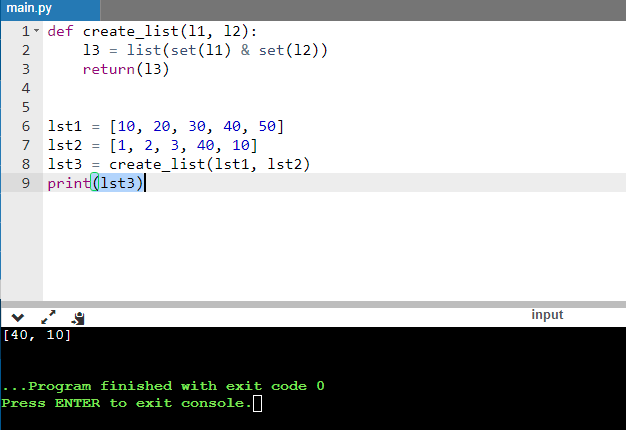# How to Write Complex Python Program Using FunctionsHere is an example of complex python program written using functions. Many times, in job interviews, you need to give a written test. There, you may need to answer tricky programs. Historically, people are afraid to take a test. Especially in python

## Complex Python programs

Below are the Complex Python Program Using Functions and examples of how to write the code.
1. Counting lower and upper case letters
2. Creating a list

## 1. Counting lower and upper case letters

Below program counts the upper and lower case letters.

def count_lower_upper(s): dlu = {'Lower': 0, 'Upper': 0} for ch in s: if ch.islower(): dlu['Lower'] += 1 elif ch.isupper(): dlu['Upper'] += 1 return(dlu) d = count_lower_upper('James BOnd') print(d) d = count_lower_upper('Anant Amrut Mahalle') print(d)Also read: How to Lose Your Weight

## 2. Creating a List

Here it uses two input lists for creating a new list. Additionally, it uses the list built-in function.

def create_list(l1, l2): l3 = list(set(l1) & set(l2)) return(l3) lst1 = [10, 20, 30, 40, 50] lst2 = [1, 2, 3, 40, 10] lst3 = create_list(lst1, lst2) print(lst3)Related